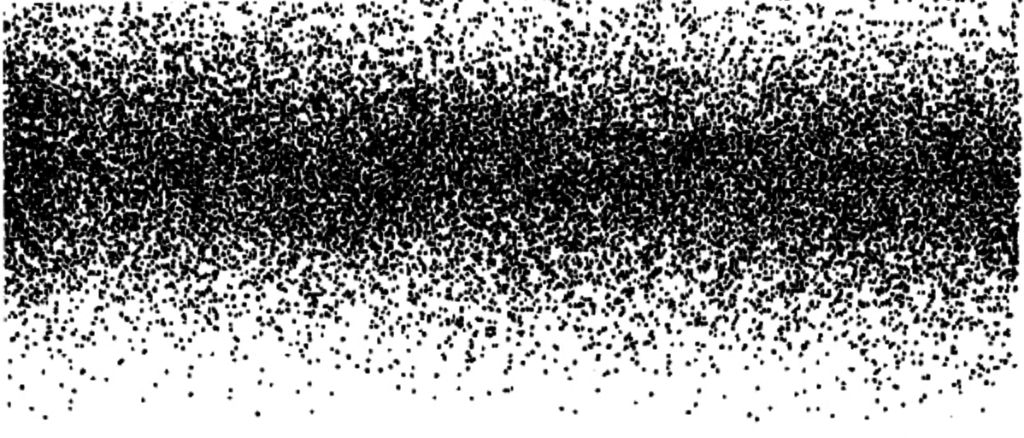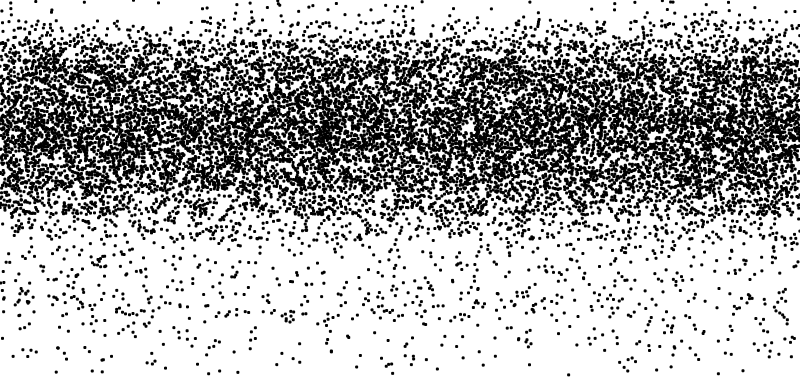# Dots dots dots

This week’s assignment was to recreate one of some older computer arts from the Triangulation webpage. I decided to make the Gaussian Distribution one – which seemed simple at first but turned out to be quite challenging to replicate. It’s just a bunch of dots distributed in different densities- well yeah, but… maybe not for me.

My first instinct was that all the dots are densely located around a horizontal line and gradually decrease density the further you get from the line. Yet I failed spectacularly trying to approach this logic – also rethinking that now, it would not reflect a small degree of wavy imperfection in the distribution.

Plan B was to define certain segments of the background to which I will assign a certain number of dots. Through layering them on each other and overlapping them I could imitate the distribution effect – though not perfectly. I played around with different segment sizes and numbers, as well as the number of dots in each.

The problem I encountered using this approach, was that the segments started showing in straight almost fully defined lines, which caused the gradual distributive effect to disappear. To “blend” these segments more I was thinking of defining them within a curvy segment rather than a rectangular one. Which worked in theory, but I could not make it work by myself. Yay.

Therefore I tried blending it through decreasing the dot number but increasing the number of segments.  Not perfect, but the best I could do. Fake it till you make it does not always apply.

This is the original version:And this is my replication:In addition, I also played a little bit with interaction. I was interested in what happens when I delete the noLooop() command, and the result made me feel actually really uncomfortable- a bunch of tiny little objects moving quickly and uncontrollably. It almost reminded me of a hive of bees.

That’s why I made the highest concentration of dots to follow the mouse cursor (similarly to what bees do) and if the space bar is pressed, the number, as well as the size of dots, start increasing until they pretty much consume the whole screen.

Sound on for an extra experience:

And last but not least, the code:

```//setting up the number of dots used in different segments
int numberCirclesA = 700;
int numberCirclesB = 1000;
int numberCirclesC = 3000;
int numberCirclesD = 5000;

//setting up variables for x, y locations, and width of the dots
float x;
float y;
float w = 3;

void setup() {
size (800, 390);
//noLoop(); // to be activated only to see the exact replica of the original image
}

void draw () {
background (255);
noStroke();
fill(0);

//if the space key is pressed then the number of circles and their size increase
if (keyPressed) {
if (key == ' ') {
w = w + .5;
numberCirclesA = numberCirclesA + 2000 ;
numberCirclesB =   numberCirclesB + 1000;
numberCirclesC =   numberCirclesC + 1000;
numberCirclesD =   numberCirclesD + 1000;
}
} else {
w = 3;
numberCirclesA = 700;
numberCirclesB = 1000;
numberCirclesC = 3000;
numberCirclesD = 5000;
}

// different segments in which the dots are being drawn

//mouseY is added to the Y location to make the segment follow the mouse cursor

for (int i = 0; i < numberCirclesA; i++) {
pushMatrix();
x = random(0, width);
y =random(0, height-15);
ellipse (x, y, w, w);
popMatrix();
}
for (int i = 0; i < numberCirclesB; i++) {
pushMatrix();
y = (random(110, 150) + mouseY);
x = (random(0, width));
ellipse(x, y, w, w);
popMatrix();
}
//
for (int i = 0; i < numberCirclesA; i++) {
pushMatrix();
x = random(0, width);
y = (random(0, height -30)+ mouseY);
ellipse (x, y, w, w);
popMatrix();
}

for (int i = 0; i < numberCirclesB; i++) {
pushMatrix();
y = (random(40, height - 70) + mouseY);
x = random(0, width);
ellipse(x, y, w, w);
popMatrix();
}

for (int i = 0; i < numberCirclesC; i++) {
pushMatrix();
y = (random(20, height - 150)+ mouseY);
x = random(0, width);
ellipse(x, y, w, w);
popMatrix();
}

for (int i = 0; i < numberCirclesD; i++) {
pushMatrix();
y = (random(60, height - 200)+ mouseY);
x = random(0, width);
ellipse(x, y, w, w);
popMatrix();
}
for (int i = 0; i < numberCirclesD; i++) {
pushMatrix();
y = (random(40, height - 175) + mouseY);
x = random(0, width);
ellipse(x, y, w, w);
popMatrix();
}

//tracking the mouser cursor
print(mouseX);
print (" ");
println(mouseY);
}
```